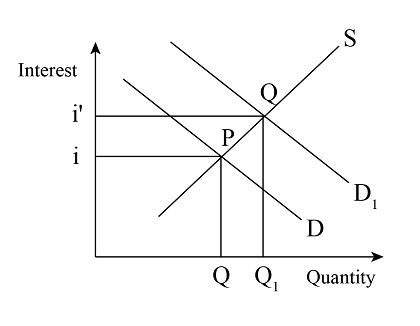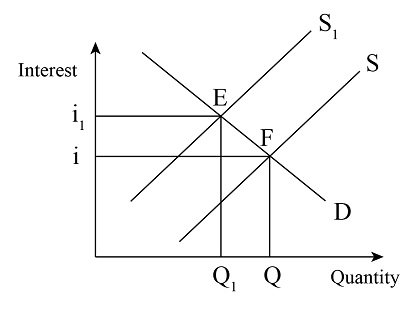# 1. Using the loanable funds model, graphically illustrate and indicate the impact on i and q if...

## Question:

1. Using the loanable funds model, graphically illustrate and indicate the impact on i and q if the U.S. Government cuts the corporate profits tax while at the same time cutting tax credits to households (making it revenue neutral for the U.S. Government).

2. Using the loanable funds model, graphically illustrate and explain the impact on i and q resulting from an increase in inflation expectations from 4.5% to 14%.

## Profit:

Profit is the extra amount of money that is earned by carrying out a regular business activity. It is calculated using the total revenue and the total cost. When the total revenue is greater than the total cost then the profit is positive.

When the government cuts the corporate profit tax, it leads to a rise in the profits of the firm and they will produce more output for which the firms will raise the demand for investment. Therefore, this will cause a rise in the demand for loanable funds and the demand curve will shift to the right.In the diagram, initially, the demand and the supply curve of loanable funds are equal at point P. The demand curve rises from D to D1and the equilibrium moves from point P to Q and the interest rate rises from i to i1. The quantity of loanable funds also increases from Q to Q1.

An increase in inflation will decrease the purchasing power of money. Hence, if the households expect that the inflation rate will increase in the future, then they will expect that the purchasing power will decline. So they will raise their current consumption which will decrease the savings. Therefore, the supply of loanable funds will decrease and this will shift the supply curve to the left.In the diagram, initially, the demand and the supply curve of loanable funds are equal at point F. The supply curve will shift from S to S1 and the equilibrium will shift from point F to E and the interest rate will rise from i to i1 and the quantity of loanable funds would decline from Q to Q1